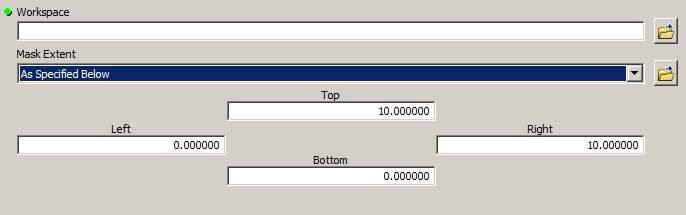# Passing user-defined extent from Custom Toolbox

956
2
09-22-2016 10:17 AMNew Contributor

I am developing an ArcPy tool that will enable a user to define a custom mask extent, which will be converted to a constant value raster and then used as input for a Zonalstats operator. I have the extent parameter added to the menu, but need some help in passing those values back to the script. The snippet of code below shows the context. The values from the menu for 'Mask Extent' have to populate Extent(xmin, ymin, xmax, ymax). I have tried setting the returned values with a GetParameterAsText, but that doesn't seem to work. Any help appreciated.

# Set local variables
constantValue = 255
cellSize = 1
outExtent = Extent(200, -800, 600, -400)2 RepliesNew Contributor

For anyone interested, the following code solved this problem.

``````mask = arcpy.GetParameterAsText(3)
MinX, MinY, MaxX, MaxY = mask.split( " ")
MinX = float( MinX)
MinY = float( MinY)
MaxX = float( MaxX)
MaxY = float( MaxY)‍‍‍‍‍‍‍‍‍‍‍‍``````

The variables were then passed as follows:

``````outExtent = Extent(MinX, MinY, MaxX, MaxY)

outConstRaster = CreateConstantRaster(constantValue, "INTEGER",
cellSize, outExtent)‍‍‍‍‍‍‍‍‍‍‍‍``````byMVP Esteemed Contributor

I don't see what the problem is here. this should work:

``````import arcpy
arcpy.CheckOutExtension("spatial")
from arcpy.sa import *

# ...

cellsize = arcpy.GetParameterAsText(2)
outExtent = arcpy.GetParameterAsText(3)
outConstRaster = CreateConstantRaster(
"1", "INTEGER",
cellSize, outExtent)‍‍‍‍‍‍‍‍‍‍‍‍‍‍‍‍‍‍‍‍‍‍‍``````